CBSE Class 10 Sample Paper for 2023 Boards - Maths Standard

Class 10
Solutions of Sample Papers for Class 10 Boards

## A train covered a certain distance at a uniform speed. If the train would have been 6 km/h faster, it would have taken 4 hours lessthan the scheduled time. And, if the train were slower by 6 km/hr ; it would have taken 6 hours more than the scheduled time. Find the length of the journey.

This question is Similar to Ex.3.7 (Optional) - Chapter 3 Class 10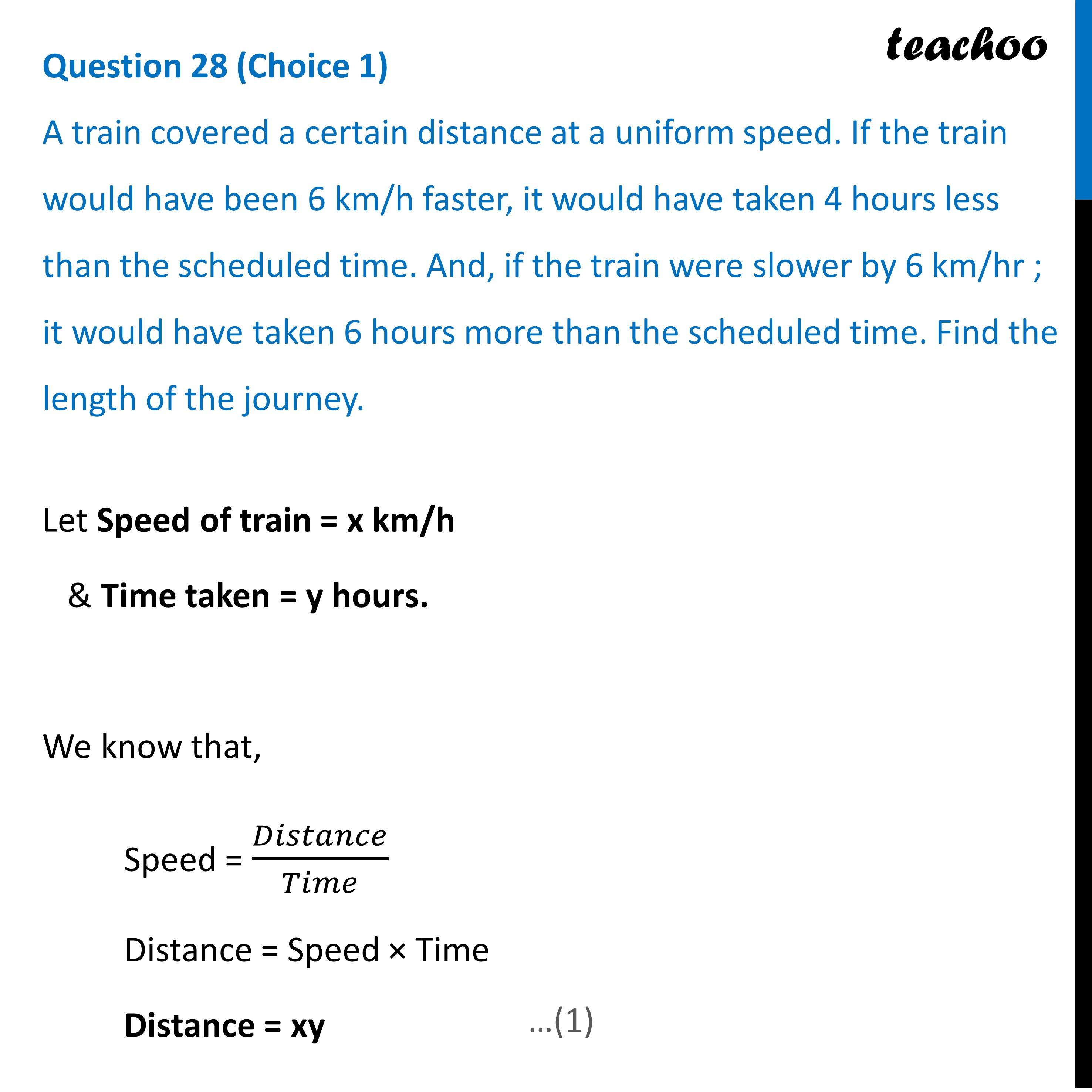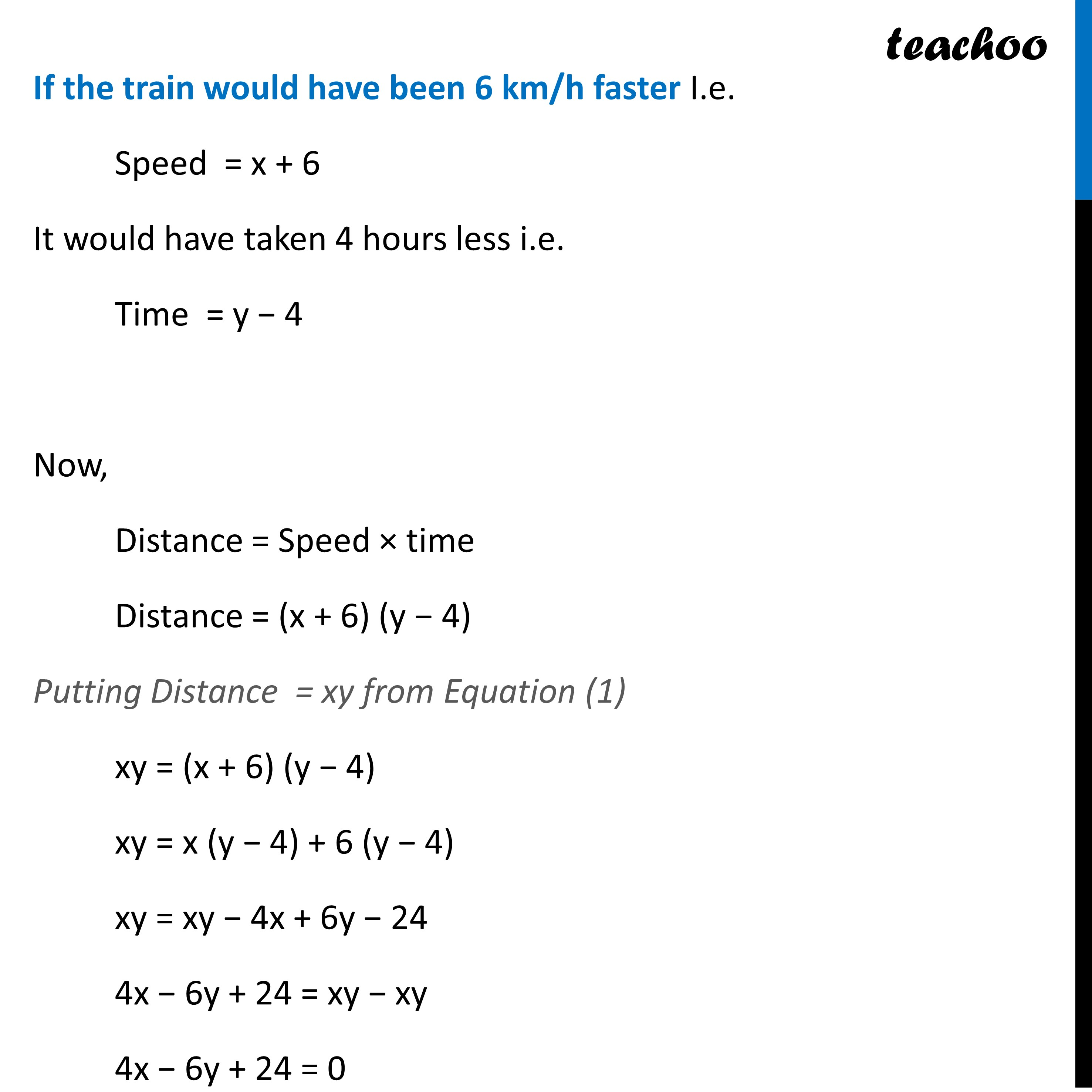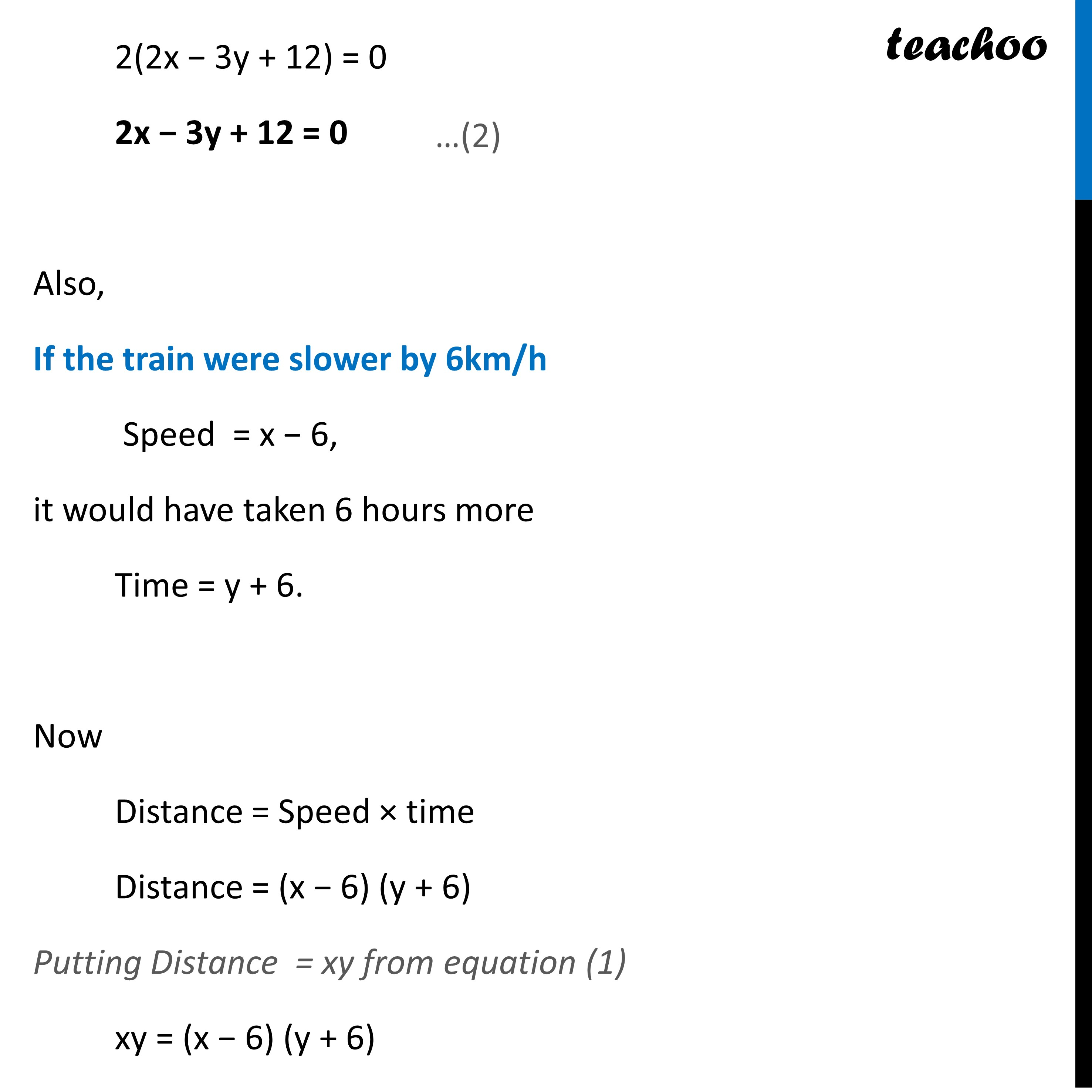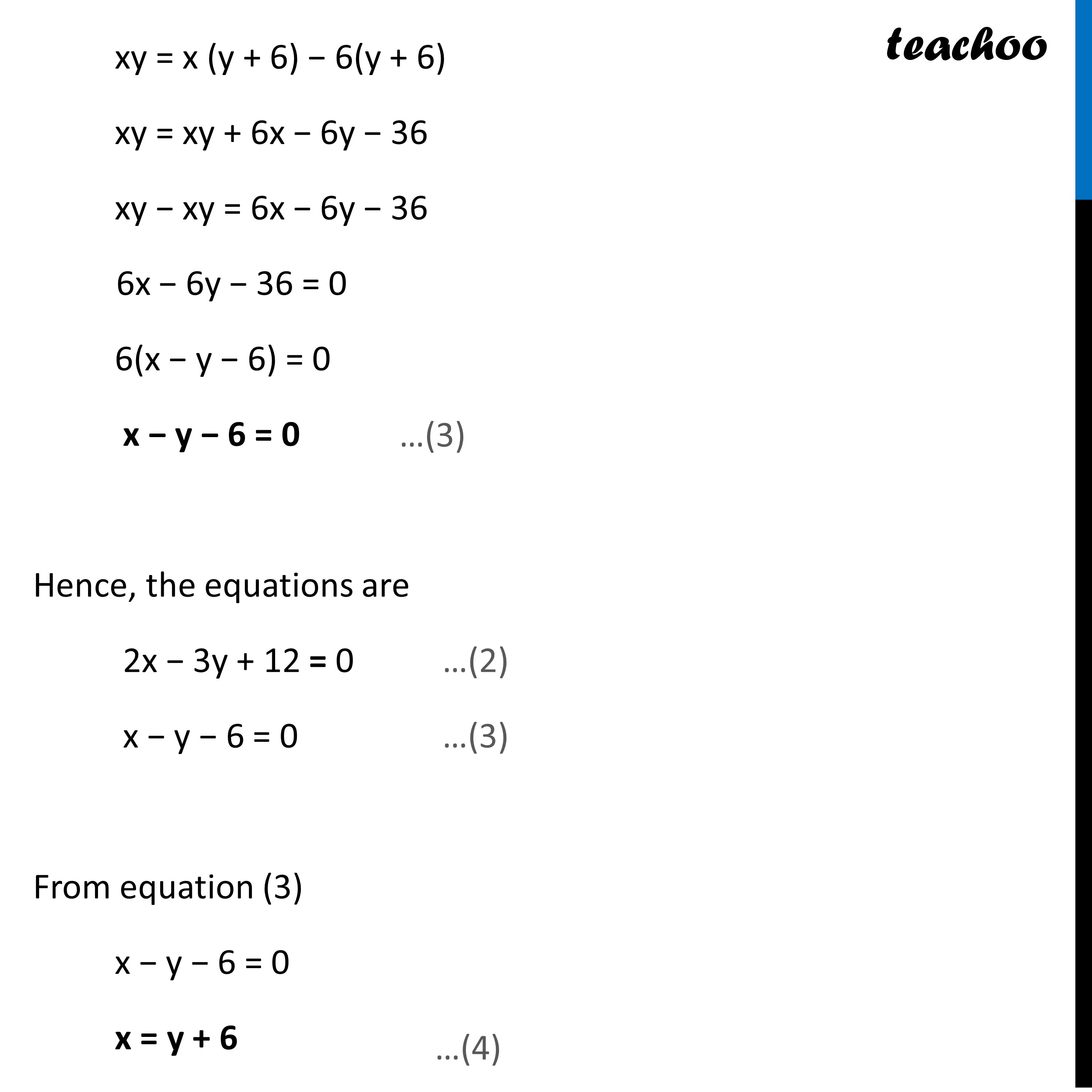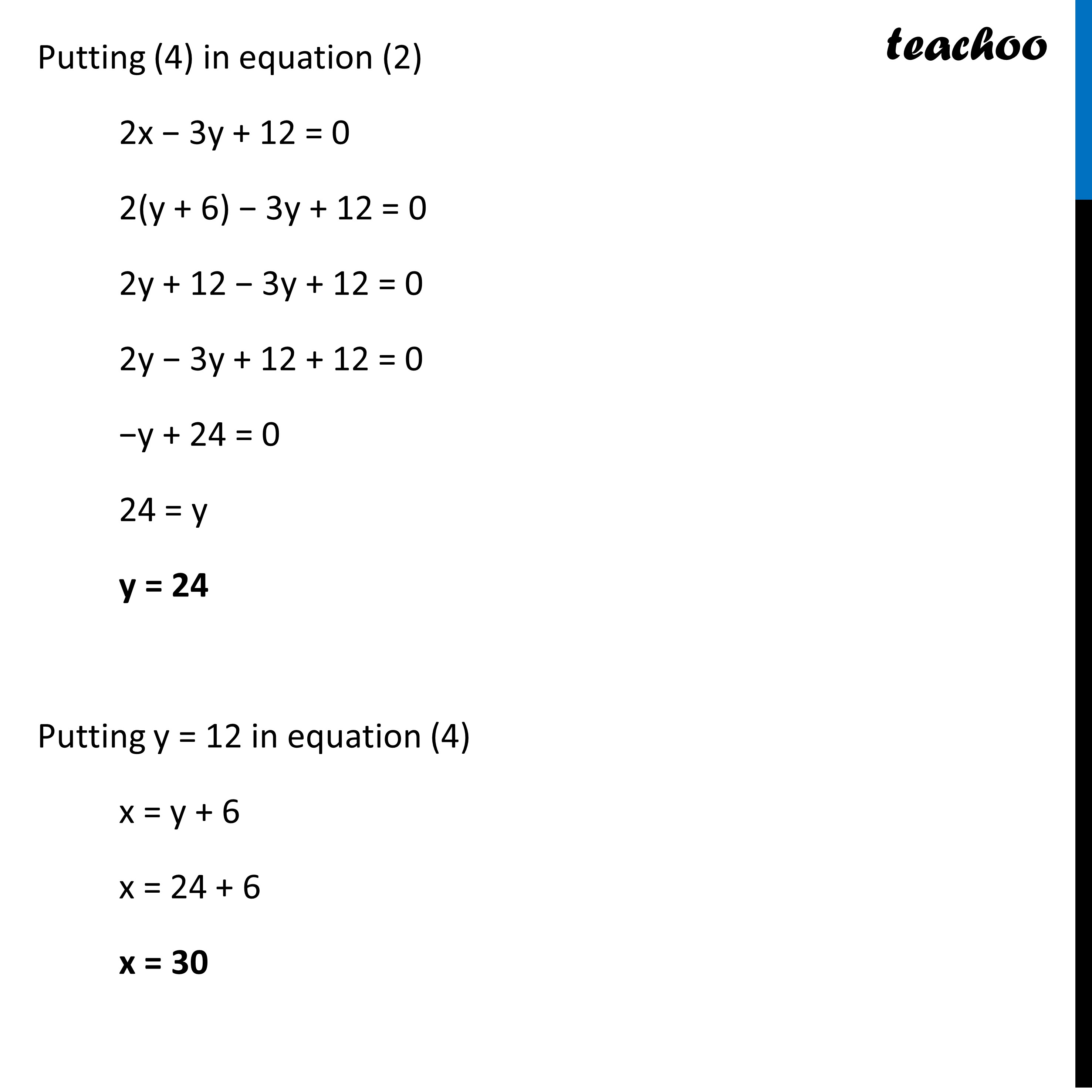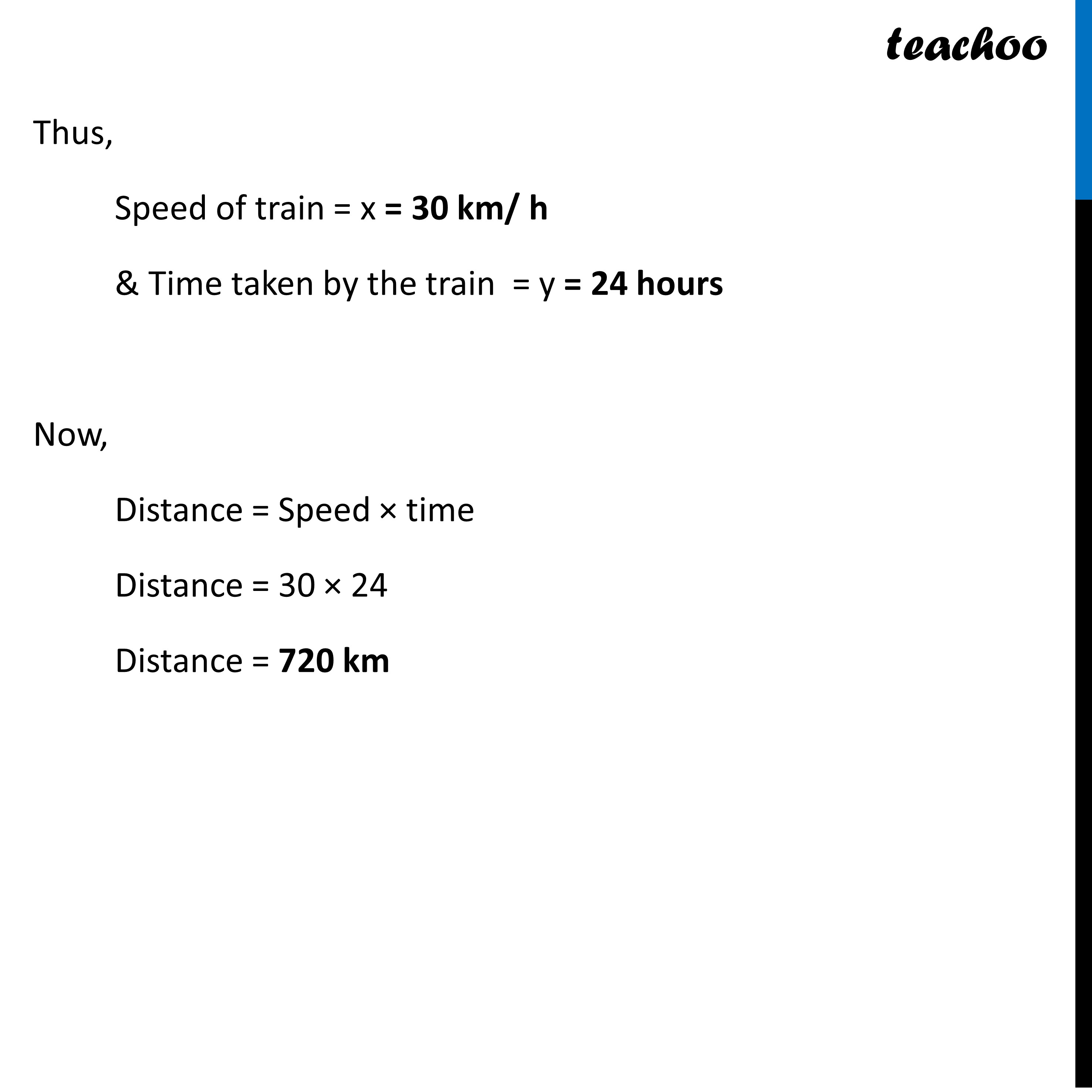Learn in your speed, with individual attention - Teachoo Maths 1-on-1 Class

### Transcript

Question 28 (Choice 1) A train covered a certain distance at a uniform speed. If the train would have been 6 km/h faster, it would have taken 4 hours less than the scheduled time. And, if the train were slower by 6 km/hr ; it would have taken 6 hours more than the scheduled time. Find the length of the journey. Let Speed of train = x km/h & Time taken = y hours. We know that, Speed = 𝐷𝑖𝑠𝑡𝑎𝑛𝑐𝑒/𝑇𝑖𝑚𝑒 Distance = Speed × Time Distance = xy If the train would have been 6 km/h faster I.e. Speed = x + 6 It would have taken 4 hours less i.e. Time = y − 4 Now, Distance = Speed × time Distance = (x + 6) (y − 4) Putting Distance = xy from Equation (1) xy = (x + 6) (y − 4) xy = x (y − 4) + 6 (y − 4) xy = xy − 4x + 6y − 24 4x − 6y + 24 = xy − xy 4x − 6y + 24 = 0 2(2x − 3y + 12) = 0 2x − 3y + 12 = 0 Also, If the train were slower by 6km/h Speed = x − 6, it would have taken 6 hours more Time = y + 6. Now Distance = Speed × time Distance = (x − 6) (y + 6) Putting Distance = xy from equation (1) xy = (x − 6) (y + 6) xy = x (y + 6) − 6(y + 6) xy = xy + 6x − 6y − 36 xy − xy = 6x − 6y − 36 6x − 6y − 36 = 0 6(x − y − 6) = 0 x − y − 6 = 0 Hence, the equations are 2x − 3y + 12 = 0 …(2) x − y − 6 = 0 …(3) From equation (3) x − y − 6 = 0 x = y + 6 Putting (4) in equation (2) 2x − 3y + 12 = 0 2(y + 6) − 3y + 12 = 0 2y + 12 − 3y + 12 = 0 2y − 3y + 12 + 12 = 0 −y + 24 = 0 24 = y y = 24 Putting y = 12 in equation (4) x = y + 6 x = 24 + 6 x = 30 Thus, Speed of train = x = 30 km/ h & Time taken by the train = y = 24 hours Now, Distance = Speed × time Distance = 30 × 24 Distance = 720 km